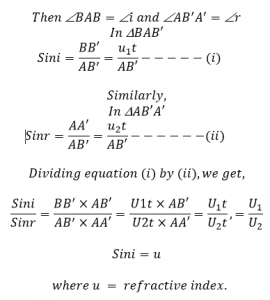# Laws Of Refraction Of Light According To The Wave Theory

According to the laws of refraction of light

The ratio of sine of angle of incidence to the sine of angle of refraction is equal to the constant value, which is called refractive index of a medium in which light bends. This law is called snell’s law.
The incident ray, refracted ray and normal lie on the same plane.
Proof:
Consider on incident wave front AB is incident towards the reflecting surface XY making an angle of incidence ‘i’ as in figure. Let v and v be the velocities of light in medium 1 and medium 2 respectively. The lines, which are drawn perpendicular to the incident wave front are incident rays i.e. a, b, and c are incident rays. According to the Huygen’s principle, every point of wave front acts as the source of secondary wave lets. Then at any time ‘t’ the point B’ of AB forms the secondary wavelets, such that whose surface just touches the point B of refracting surface XY and BB’= U1T. In the same time, the point A’ forms the secondary wavelets. Such that AA= U2T parallel. Another point of AB forms the secondary wavelets, such that the sum of the tangents drawn to the surface of these secondary wavelets give the new position of wave front .ie A’B’ is refracting wave front which makes an angle of refraction r. The line, which are drawn perpendicular to the refracting wave front i.e. a’, b’ and c’ are refracted ray.

Since i and r are angle of incidence and angle of refraction,This proves snell’s law.

Since incident wave front, refracting surface and refracted wave front are perpendicular to the plane of paper. Then incident ray. Normal and refracted ray which are perpendicular to the incident to the wave front, refracted surface and refracted wave front are parallel to the plane of paper. Hence they lie on the same plane which proves the laws of refraction of light.